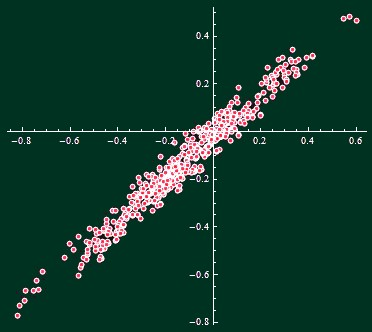## Tuesday, October 05, 2010 ...//### The likelihood that 2010 will surpass 1998

It's time for some climate change facts. This text is just an update of two articles written three months ago and one month ago into which I will add one additional month of data and refresh all the numbers.

The nearly final temperature readings of UAH AMSU for September 2010 are out and the global temperature anomaly was 0.60 °C, higher than 0.51 °C a month earlier.So September 2010 was 0.15 °C warmer (thanks, Ivan!) than September 1998 and it is just a slightly-above-average month of 2010 so far. Also, the average anomaly of the first nine months (Part1) of 2010, 0.55 °C, is cooler than Part1 of 1998, 0.59 °C, by 0.04 °C. Of course, if this temperature difference continued for the last three months (Part2) of 2010, 2010 would be cooler than 1998.But Part2 is different from Part1, so this simple extrapolation isn't possible. You may ask: how likely it is for 2010 to be cooler than 1998 if we know that Part1 of 2010 was 0.04 °C cooler than Part1 of 1998? To answer this question statistically, let's simply look at 31 years of the UAH AMSU record, 1979-2009.

We may look at (31 choose 2) = 465 pairs of years. For each of them, we may calculate the difference of the average temperatures of their first 8 months, Part1, and the difference of the average annual temperatures. The Part1 differences will be shown on the x-axis and the annual (3 Part1 + Part2)/4 differences will appear on the y-axis of the chart below:A pretty nice, straight line with a pretty nice, normal "noisy" distribution away from the line itself. It's of course more straight than what we obtained one month and especially three months ago: our ability to predict the average of 2010 is getting better as we approach the last month. Linear regression gives

yearDifference = -0.023 + 0.89 h1Difference
Note that the slope, 0.89, is positive but smaller than one (but closer than one than in previous months). We may say that while Part2 tends to be correlated with Part1, Part2-Part1 is actually negatively correlated with Part1 itself, so Part2 acts to reduce the temperature difference between two years in most cases.

Now, the individual data points deviate from the idealized line. What is the root mean square of the differences? The answer turns out to be 0.044 °C (less than 0.073 °C we got three months ago and 0.055 °C one month ago). This is the "thickness" of the line in the chart above in the y-direction.

It's enough for us to make a prediction for 2010, using the comparison with 1998.

The Part1 was 0.0389 °C cooler than in 1998. Multiply it by the slope, 0.89, to see that the whole year 2010 is predicted to be 0.034 °C cooler than the year 1998. At least, that's the central value. However, this predicted difference has some normally distributed noise whose standard deviation is 0.044 °C, as we said.

That means that the predicted difference is 0.79 standard deviations (0.034/0.044). Use the error function, or (approximately) this graph,to see that the probability that 2010 will still end up being warmer than 1998, according to UAH AMSU, is about
P = 21 percent.
The formula in Mathematica is (1 + Erf[-0.79/Sqrt])/2. The odds are about 4-to-1 that 2010 will stay cooler than 1998. Note that because September 2010 didn't manage to cool the Earth - while in 1998, September already indicated the big cooling after the El Nino of the century - the probability that 2010 will be warmer than 1998 in the UAH books has increased since what it was one month ago.

Four is kind of greater than one - but it is not "overwhelmingly" greater - and 21% is a typical probability when you have to say that "it is too close to call". Moreover, if the 2010-1998 temperature difference in the last three months of the year stayed the same what it was in September - and it was high, 0.15 °C - then 2010 could possibly beat 1998. So the true likelihood may be around 50 percent rather than 21 percent. Whether the strengthening La Nina will manage to be heard in the global UAH temperature data before 2010 ends is yet to be seen.

This time, I will omit the alternative ways to predict 2010 from a smaller (or weighted) ensemble of the months.